# A parameter-dependent refinement of the discrete Jensen's inequality for convex and mid-convex functions

## Abstract

In this paper, a new parameter-dependent refinement of the discrete Jensen's inequality is given for convex and mid-convex functions. The convergence of the introduced sequences is also studied. One of the proofs requires an interesting convergence theorem with probability theoretical background. We apply the results to define some new quasi-arithmetic and mixed symmetric means and study their monotonicity and convergence.

## 1 Introduction and the main results

The considerations of this paper concern

(A1) an arbitrarily given real vector space X, a convex subset C of X, and a finite subset {x1,..., x n } of C, where n ≥ 1 is fixed;

(A2) a convex function f : C, and a discrete distribution p1,..., p n , which means that p j ≥ 0 with;

(A3) a mid-convex function f : C, and a discrete distribution p1,..., p n with rational p j (1 ≤ jn).

The function f : C is called convex if(1)

and mid-convex ifFor a variety of applications, the discrete Jensen's inequalities are important:

Theorem A. (see ) (a) If (A1) and (A2) are satisfied, then(2)

(b) If (A 1 ) and (A 3 ) are satisfied, then (2) also holds.

Let := {0, 1, 2,...} and let + := {1, 2,...}.

Various attempts have been made to refine inequality (2) in the following ways: Assume either (A1) and (A2) or (A1) and (A3). Let m ≥ 2 be an integer, and let I denote either the set {1,..., m} or the set +.

(B) Create a decreasing real sequence (B k )kIsuch that B k = B k (f, x i , p i ) (k I) is a sum whose index set is a subset of {1,..., n} k and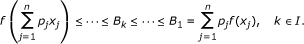(3)

(C) Create an increasing real sequence (C k )kIsuch that C k = C k (f, x i , p i ) (k I) is a sum whose index set is a subset of {1,..., k}nand(4)

The next two typical results belong to the group of refinements of type (B).

These examples use. In , Pečarić and Volenec have constructed the sequence(5)

while the other sequence(6)

is due to Pečarić and Svrtan . In a recent work,  Horváth and Pečarić define a lot of new sequences, they generalize and give a uniform treatment a number of well-known results from this area, especially (5) and (6) are extended. Horváth develops a method in  to construct decreasing real sequences satisfying (3). His paper contains some improvements of the results in  and gives a new approach of the topic. The description of the sequences in [4, 5] requires some work, so we do not go into the details. The problem (B) has been considered for the classical Jensen's inequality by Horváth .

We turn now to the group of refinements of type (C). In contrast to the previous problem, it is not easy to find such results. Recently, Xiao et al.  have obtained the sequence(7)

which satisfies (4) with.

In this paper, we establish a new solution of the problem (C). The constructed sequence (C k (λ))k≥0depends on a parameter λ belonging to [1, ∞[, and we can use arbitrary discrete distribution p1,..., p n , not just the appropriate discrete uniform distribution. We give the limit of the sequence under fixed parameter. We also study the convergence of the sequence when the parameter varies and k is fixed. Finally, some applications are given which concern the theme of means.

The next theorems are the main results of this paper. We need some further hypotheses:

(A4) Let λ ≥ 1.

(A5) Let λ ≥ 1 be rational.

First, we give a refinement of the discrete Jensen's inequality (2).

Theorem 1 Suppose either (A 1 ), (A 2 ), and (A 4 ) or (A 1 ), (A 3 ), and (A 5 ). Introduce the setsand for k define the numbers(8)

Then,Remark 2 (a) It follows from the definition of S k that S k {0,..., k} n (k ).

(b) It is easy to see that(9)

Finally, we establish two convergence theorems.

Theorem 3 Suppose (A1), (A2), and (A4). Suppose × is a normed space and f is continuous. Then,

(a) For every fixed λ > 1(b) The function λC k (λ) (λ ≥ 1) is continuous for every k .

The proof of Theorem 3(a) requires a lemma (see Lemma 15), which is interesting in its own right. Probability theoretical technique will be used to handle this problem.

Remark 4 In the previous theorem, it suffices to consider the case when (A1), (A2), and (A4) are satisfied. Really, if f is mid-convex and continuous, then convex.

By (9)We come now to the second convergence theorem.

Theorem 5 Suppose either (A1), (A2), and (A4) or (A1), (A3), and (A5). For each fixed k +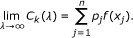## 2 Discussion and applications

Suppose either (A1), (A2), and (A4) or (A1), (A3), and (A5). First, we give three special cases of (8).

(a) k = 1, n +:(b) k , n = 2:(c):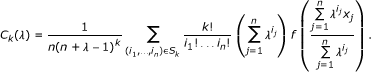Assume further that f is strictly convex (strictly mid-convex) that is strict inequality holds in (1) whenever xy and 0 < α < 1. In this case, equality is satisfied in (2) if and only if x1 = ··· = x n , and therefore, it comes from the third part of the proof of Theorem 1 that(10)

if not all x i are equal.

Ifand f is strictly convex (strictly mid-convex), then the analysis of the proof of Theorem 1 shows thatwhenever not all x i are equal.

If the inequality (10) holds, X is a normed space and f is continuous (see Remark 4), then Theorem 3(b) and Theorem 5 insure that the range of the function λC k (λ) (k +) is the intervalConjecture 6 Suppose either (A1), (A2), and (A4) or (A1), (A3), and (A5).

The function λC k (λ) (λ ≥ 1) is increasing for every k .

Next, we define some new quasi-arithmetic means and study their monotonicity and convergence. About means see .

Definition 7 Let I be an interval, let x j I (1 ≤ jn), let p1,..., p n be a discrete distribution, and let g, h : I be continuous and strictly monotone functions. Let λ ≥ 1. We define the quasi-arithmetic means with respect to (8) by(11)

Some other means are also needed.

Definition 8 Let I be an interval, let x j I (1 ≤ jn), and let p1,..., p n be a discrete distribution. For a continuous and strictly monotone function z : I, we introduce the following mean(12)

We now prove the monotonicity of the means (11) and give limit formulas.

Proposition 9 Let I be an interval, let x j I (1 ≤ jn), let p1,..., p n be a discrete distribution, and let g, h : I be continuous and strictly monotone functions. Let λ ≥ 1. Then,

(a)if either h g-1is convex and h is increasing or h g-1is concave and h is decreasing.

(b)if either h g-1is convex and h is decreasing or h g-1is concave and h is increasing.

(c) Moreover, in both casesfor each fixed λ > 1, andfor each fixed k +.

Proof. Theorem 1 can be applied to the function hg-1, if it is convex (-hg1, if it is concave) and the n-tuples (g (x1),..., g(x n )), then upon taking h-1, we get (a) and (b). (c) comes from Theorems 3(a) and 5. ■

As a special case, we consider the following example.

Example 10 If I :=]0, ∞[, h := ln, and g(x):= x (x ]0, ∞[), then by Proposition 9(b), we have the following inequality. for every x j > 0 (1 ≤ jn), λ ≥ 1 , and k +which gives a sharpened version of the arithmetic mean - geometric mean inequalityFinally, we investigate some mixed symmetric means.

The power means of order r are defined as follows:

Definition 11 Let x j ]0, ∞[ (1 ≤ jn), and let p1,..., p n be a discrete distribution with p j > 0 (1 ≤ jn).If r ≠ 0, then the power means of order r belong to the means (12) (z : ]0, ∞[→ , z(x) := xr ), while we get the power means of order 0 by taking limit. Supported by the power means, we can introduce mixed symmetric means corresponding to (8):

Definition 12 Let x j ]0, ∞[ (1 ≤ jn), and let p1,..., p n be a discrete distribution with p j > 0 (1 ≤ jn). Let λ ≥ 1, and k . We define the mixed symmetric means with respect to (8) byif s, t and s ≠ 0, andwhere t .

The monotonicity and the convergence of the previous means are studied in the next result.

Proposition 13 Let x j ]0, ∞[ (1 ≤ jn), and let p1,..., p n be a discrete distribution with p j > 0 (1 ≤ jn). Let λ ≥ 1, and k . Suppose s, t such that st. Then,

(a)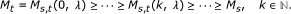(b) In case of s, t ≠ 0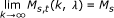for each fixed λ > 1, andfor each fixed k +.

Proof. Assume s, t ≠ 0. Then, Proposition 9 (b) can be applied with g, h :]0, ∞[→ , g(x) := xt , and h(x) := xs . If s = 0 or t = 0, the result follows by taking limit. ■

## 3 Some lemmas and the proofs of the main results

Lemma 14 Let k and (i1,..., i n ) Sk+1be fixed. If we set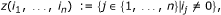thenProof. The lowest common denominator is i1!... i n !. Combined with, the result follows. ■

The proof of Theorem 1.

Proof. (a) We separate the proof of this part of the theorem into three steps.

Let λ ≥ 1 be fixed.

I. Since S 0 = {(0,..., 0)}II. Next, we prove that C k (λ) ≤ C k+1(λ) (k ).

It is easy to check that for every (i1,..., i n ) S kWith the help of Theorem A, this yields thatConsequently,(13)

By Lemma 14, it is easy to see that the right-hand side of (13) can be written in the form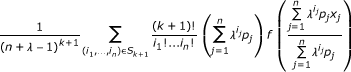which is just Ck+1(λ).

III. Finally, we prove that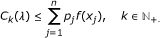(14)

It follows from Theorem A that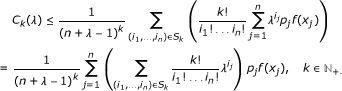(15)

The multinomial theorem shows that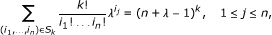hence (15) implies (14). ■

The proof of Theorem 3 (a) is based on the following interesting result. The σ-algebra of Borel subsets of n is denoted by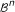.

Lemma 15 Let p1,..., p n be a discrete distribution with n ≥ 2, and let λ > 1. Let l {1,..., n} be fixed. e l denotes the vector in n that has 0s in all coordinate positions except the lth, where it has a 1. Let q1,..., q n be also a discrete distribution such that q j > 0 (1 ≤ jn) and(16)

Ifis a bounded function for whichexists in and p l > 0, then(17)

Proof. To prove the result, we can obviously suppose that l = 1.

For the sake of clarity, we shall denote the element (i1,..., i n ) of S k by (i1k,..., i nk ) (k +).

Let ξ k := (ξ1k,..., ξ nk ) (k +) be a ( n ,)-random variable on a probability spacesuch that ξ k has multinomial distribution of order k and with parameters q1,..., q n . A fundamental theorem of the statistics (see , Theorem 5.4.13), which is based on the multidimensional central limit theorem and the Cochran-Fisher theorem, implies that(18)

where Fn-1means the distribution function of the Chi-squared distribution (χ2-distribution) with n - 1 degrees of freedom.

Choose 0 < ε < 1. Since Fn-1is continuous and strictly increasing on ]0, ∞[, there exists a unique t ε > 0 such thatDefineThe definition of the setshows that(19)(20)

where by (18)(21)

For j = 1,..., n construct the sequencesby(22)

We claim that(23)

Fix 1 ≤ jn. If (23) is false, then (22) yields that we can find a positive number ρ, a strictly increasing sequence (k u )u≥1and points(24)

such thatand therefore,contrary to (24).

LetIt follows from (16) that(25)

By (22) and (23), we can find an integer k γ such that for each k > k γThus, for every k > k γand hence, we get from (25) that(26)

We can see that(27)

Now, set, and consider the sequencesandwhere k +. The sum of these sequences is just the studied sequence in (17).

Since p1> 0, we obtain from (27) that(28)

and(29)

According to (26), the convergence is uniform for all the possible sequences in (28) and (29); hence, for every ε1> 0, we can find an integerthat for all(30)

Bringing in (19-20), we find thatand therefore, thanks to (19-20), (30) and the boundedness of g (|g| ≤ m)Consequently, by (21)and this proves the convergence claim (17).

The proof is now complete. ■

The proof of Theorem 3.

Proof. (a) We have only to observe that for every fixed 1 ≤ ln(31)

The case p l = 0 is trivial.

To prove the case p l > 0, define the functionbyConsequently, the limit in (31) can be written in the formNow, we can apply Lemma 15 withand(b) Elementary considerations show this part of the theorem.

The proof is complete. ■

The proof of Theorem 5.

Proof. Theorem A confirms that f is bounded on the setwhere t j (1 ≤ jn) is also rational if f is mid-convex.

It is elementary that for every (i1,..., i n ) S kBy the definition of the set S k , (0,..., 0, k, 0,..., 0) (the vector has 0s in all coordinate positions except the l th) is the only element of S k for which i l = k (1 ≤ ln). By using the boundedness of f on G, the previous assumptions imply the result, bringing the proof to an end. ■

## References

1. Hardy GH, Littlewood JE, Pólya G: Inequalities. Cambridge Mathematical Library Series. Cambridge University Press; 1967.

2. Pečarić JE, Volenec V: Interpolation of the Jensen inequality with some applications, Österreích. Akad Wiss Math-Natur KI Sitzungsber II 1988, 197: 463–467.

3. Pečarić JE, Svrtan D: Unified approach to refinements of Jensen's inequalities. Math Inequal Appl 2002, 5: 45–47.

4. Horváth L, Pečarić JE: A refinement of the discrete Jensen's inequality. Math Inequal Appl (to appear)

5. Horváth L: A method to refine the discrete Jensen's inequality for convex and mid-convex functions. Mathematical and Computer Modelling, in press. 10.1016/j.mcm.2011.05.060

6. Horváth L: Inequalities corresponding to the classical Jensen's inequality. J Math Inequal 2009, 3: 189–200.

7. Xiao Z-G, Srivastava HM, Zhang Z-H: Further refinements of the Jensen inequalities based upon samples with repetitions. Math Comput Mod 2010, 51: 592–600.

8. Mitrinović DS, Pečarić JE, Fink AM: Classical and New Inequalities in Analysis, vol. 61 of Mathematics and Its Applications. Kluwer Academic Publishers, Dordrecht; 1993.

9. Dacunha-Castello D, Duflo M: Probability and Statistics I. Springer-Verlag, New York; 1986.

## Acknowledgements

This study was supported by the Hungarian National Foundations for Scientific Research Grant No. K73274.

## Author information

Authors

### Corresponding author

Correspondence to László Horváth.

### Competing interests

The author declares that he has no competing interests.

## Rights and permissions

Reprints and Permissions

Horváth, L. A parameter-dependent refinement of the discrete Jensen's inequality for convex and mid-convex functions. J Inequal Appl 2011, 26 (2011). https://doi.org/10.1186/1029-242X-2011-26

• Accepted:

• Published:

• DOI: https://doi.org/10.1186/1029-242X-2011-26

### Keywords

• Normed Space
• Convergence Theorem
• Monotone Function
• Discrete Distribution
• Multinomial Distribution